# Composite of increasing functions is increasing

## Statement

### Statement for two functions

Suppose$f$ and$g$ are both functions of one variable that are increasing functions on their respective domains. Consider the composite of two functions$f \circ g$. This is also an increasing function on its domain.

Note that the statement makes no assumptions about the continuity or differentiability of the functions or even the nature of their domains. In fact, we do not even require that the domains and ranges be subsets of the real numbers, but only require that they be totally ordered sets so that the notion of increasing makes sense.

### Statement for multiple functions

Fill this in later

## Proof

### Proof for two functions

Given:$f$ and$g$ are increasing functions.$x_1 < x_2$ are both in the domain of the composite function$f \circ g$.

To prove: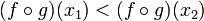$(f \circ g)(x_1) < (f \circ g)(x_2)$.

Proof:

Step no. Assertion Given data used Previous steps used Explanation
1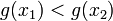$g(x_1) < g(x_2)$$g$ is increasing$x_1 < x_2$
apply definition of increasing
2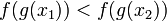$f(g(x_1)) < f(g(x_2))$$f$ is increasing Step (1) apply definition of increasing to inputs$g(x_1), g(x_2)$, use Step (1).
3$(f \circ g)(x_1) < (f \circ g)(x_2)$ Step (2) Just rewrite Step (2) in terms of composite function, using the definition of composite function.

### Compatibility with chain rule for differentiation

In case both the functions$f$ and$g$ are differentiable, then we can check that the statement is compatible with the chain rule for differentiation. In fact, the chain rule for differentiation can also be used to furnish an alternative proof in this case, though we have to deal carefully with the case of zero derivative.

Note that:$\! (f \circ g)'(x) = f'(g(x))g'(x)$

In particular

• If both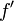$\! f'$ and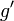$\! g'$ are positive everywhere on their domain, so is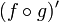$\! (f \circ g)'$.
• If both$\! f'$ and$\! g'$ are nonnegative everywhere on their domain, so is$\! (f \circ g)'$.

These almost prove that a composite of increasing differentiable functions is increasing.